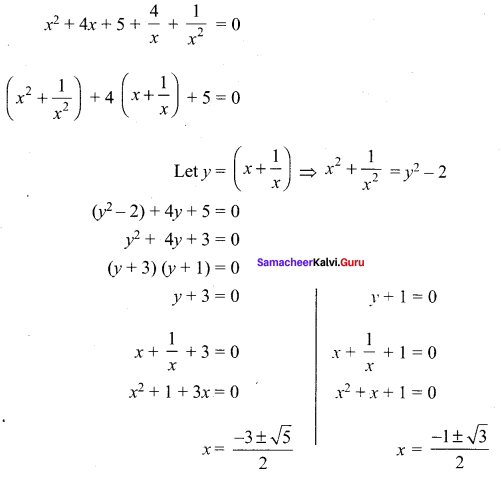# Samacheer Kalvi 12th Maths Solutions Chapter 3 Theory of Equations Ex 3.5

## Tamilnadu Samacheer Kalvi 12th Maths Solutions Chapter 3 Theory of Equations Ex 3.5

Question 1.
Solve the following equations:
(i) sin2x – 5sinx + 4 = 0
(ii) 12x3 + 8x = 29x2 – 4
Solution:
(i) sin2x – 5sinx + 4 = 0
Let y = sin x
(y2 – 5y + 4 = 0
(y – 1) (y – 4) = 0
(y – 1) = o or (y – 4) = 0
y = 1 or y = 4
sin x = 1 or sin x = 4 [not possible since sin x ≤ 1]
sin x = sin $$\frac{\pi}{2}$$
x = nπ + (-1)n α, n ∈ z.
x = nπ + (-1)n $$\frac{\pi}{2}$$
(ii) 12x3 + 8x = 29x2 – 4
12x3 – 29x2 + 8x + 4 = 0 ….. (1)
By Trail and error method, (x – 2) is a factor of (1)
The other factor is 12x2 – 5x – 2
The roots is 12x2 – 5x – 2 = 0
(3x – 2) (4x + 1) = 0
x = $$\frac{2}{3}$$, x = $$-\frac{1}{4}$$
The roots are 2, $$\frac{2}{3}$$, $$-\frac{1}{4}$$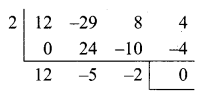[Here an = 12, a0 = 4; Let $$\frac{p}{q}$$ be the root of the equation (1)
The factors of a0 : ±1, ±2, ±4 (P must divisible by 4)
The factor of an : ±1, ±2, ±3, ±4, ±6, ±12
q must divide as (12)
Using these p and q we can form $$\pm 1, \pm 2, \pm \frac{1}{2}, \pm \frac{2}{3}, \pm \frac{1}{6}, \pm \frac{1}{4}, \pm \frac{3}{4}, \pm 3$$ are the possible roots of equation. (1)]

Question 2.
Examine for the rational roots of:
(i) 2x3 – x2 – 1 = 0
(ii) x8 – 3x + 1 = 0
Solution:
(i) 2x3 – x2 – 1 = 0
Sum of the co-efficients = 0
∴ (x – 1) is a factor
The other factor is 2x2 + x + 1.
The root is (2x2 + x + 1) = 0
Here ∆ = b2 – 4ac = (1)2 – 4(2) (1) = 1 – 8 = -7 < 0
The remaining roots are imaginary.
The only rational root is x = 1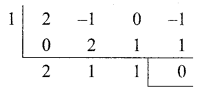(ii) x8 – 3x + 1 = 0 …. (1)
Here an = 1, a0 = 1
If $$\frac{p}{q}$$ is a rational root of (1)
Then q is a factor an, p is a factor of a0
The possible values of p and q are ± 1.
Among the possible values 1, -1, [(p, q) = 1]
None of them satisfies the equation (1)
The above equation has no rational roots.Question 3.
Solve: $$8 x^{\frac{3}{2 n}}-8 x^{\frac{-3}{2 n}}=63$$
Solution: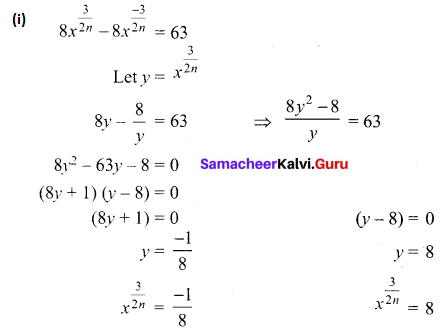Squaring on both sides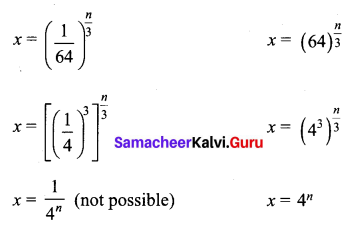only possible solution is x = 4n

Question 4.
Solve: $$2 \sqrt{\frac{x}{a}}+3 \sqrt{\frac{a}{x}}=\frac{b}{a}+\frac{6 a}{b}$$
Solution: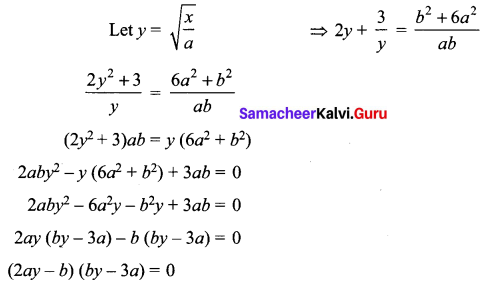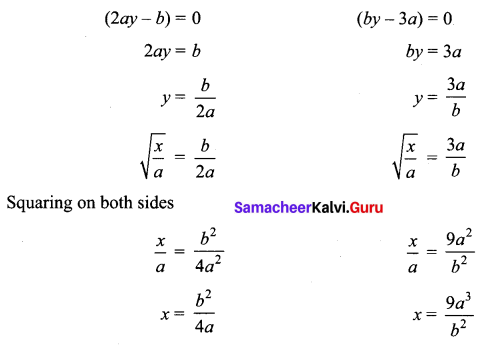Question 5.
Solve the equations:
(i) 6x4 – 35x3 + 62x2 – 35x + 6 = 0
(ii) x4 + 3x3 – 3x – 1 = 0
Solution:
(i) 6x4 – 35x3 + 62x2 – 35x + 6 = 0 ….. (1)
It is a even degree reciprocal equation as p(x) = $$x^{n} p\left(\frac{1}{x}\right)$$
Dividing equation (1) by x2,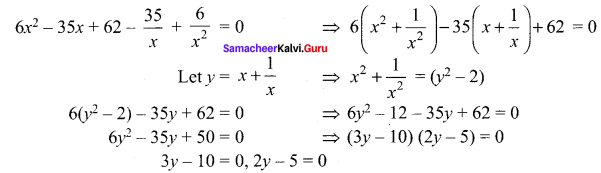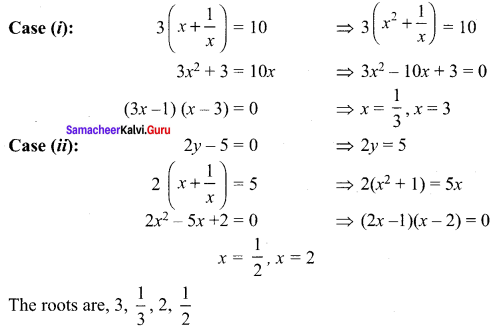(ii) x4 + 3x3 – 3x – 1 = 0 …… (1)
It is an even degree reciprocal function of type II.
1, -1 are the solution of equation (1)
(x – 1), (x + 1) are the factor of (1)
(x2 – 1) is a factor of (1)
Dividing (1) by (x2 – 1)
we get, x2 + 3x + 1 = 0 is the other factor.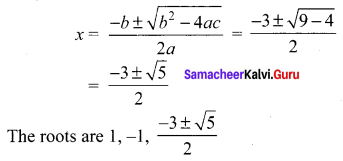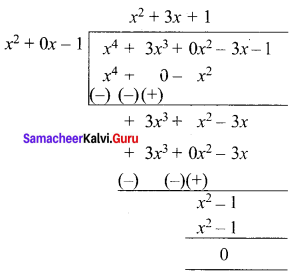Question 6.
Find all real numbers satisfying 4x – 3(2x+2) + 25 = 0
Solution:
4x – 3 (2x+2) + 25 = 0
⇒ (22)x – 3(2x . 22) + 25 = 0
(22)x – 12 . 2x+ 32 = 0
Let y = 2x
y2 – 12y + 32 = 0
⇒ (y – 4) (y – 8) = 0
y – 4 = 0 or y – 8 = 0
Case (i): 2x = 4
⇒ 2x = (2)2
⇒ x = 2
Case (ii): 2x = 8
⇒ 2x = (2)3
⇒ x = 3
∴ The roots are 2, 3Question 7.
Solve the equation 6x4 – 5x3 – 38x2 – 5x + 6 = 0 if it is known that $$\frac{1}{3}$$ is a solution.
Solution:
6x4 – 5x3 – 38x2 – 5x + 6 = 0 …… (1)
x = $$\frac{1}{3}$$ is a Solution
∴ (3x – 1) is a factor of (1)
(1) is a Reciprocal equation even degree divide (1) by x2.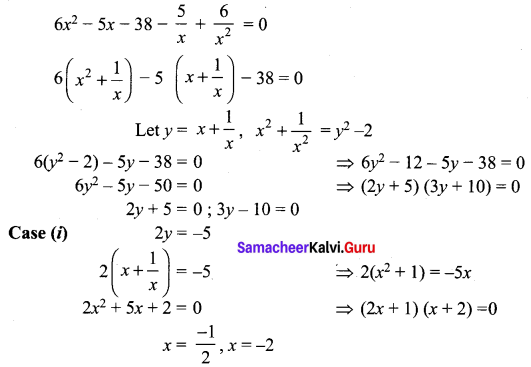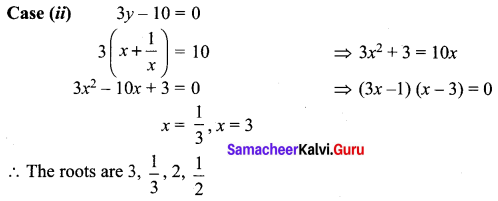### Samacheer Kalvi 12th Maths Solutions Chapter 3 Theory of Equations Ex 3.5 Additional Problems

Question 1.
Solve: 32x + 4 + 1 = 2.3x + 2
Solution:
32x + 4 = 3x + 2 + 3x + 2 – 1
32x + 4 – 3x + 2 = 3x + 2 – 1 ⇒ 3x + 2[3x + 2 – 1] = [3x + 2 – 1]
3x + 2= 1 ⇒ 3x + 2 = 30
x + 2 = 0 ⇒ x = -2

Question 2.
Solve: 2x – 2x + 3 + 24 = 0.
Solution:
22x – (2x.23) + 24 = 0 since 23 = 8 = 4 + 4 = 22 + 22
22x – (4 + 4)2x + 24 = 0 ⇒ 22x – (22 + 22)2x + 24 = 0
(2x – 22)(2x – 22) = 0 ⇒ (2x – 22)2 = 0
2x = 22 ⇒ x = 2

Question 3.
Solve: (x – 4) (x + 2) (x + 3) (x – 3) + 8 = 0.
Solution:
(x – 4) (x + 3) (x + 2) (x – 3) + 8 = 0
(x2 – x – 12) (x2 – x – 6) + 8 = 0
Let y = x2 – x
(y – 12) (y – 6) + 8 = 0 ⇒ y2 – 18y + 72 + 8 = 0
y2 – 18y + 80 = 0 ⇒ (y – 10)( y – 8) = 0
Case (i) y – 10 = 0
x2 – x – 8 = 0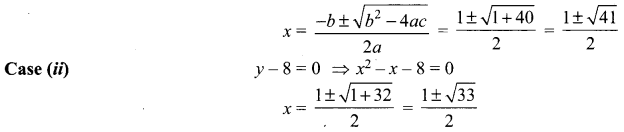Question 4.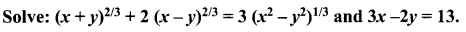Solution: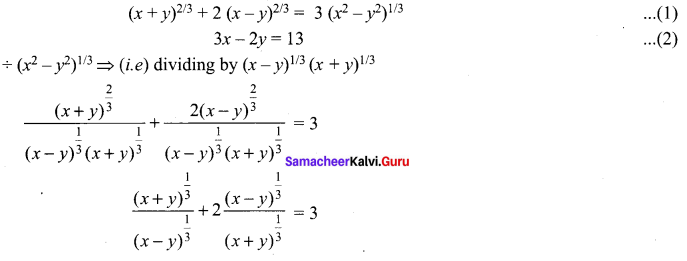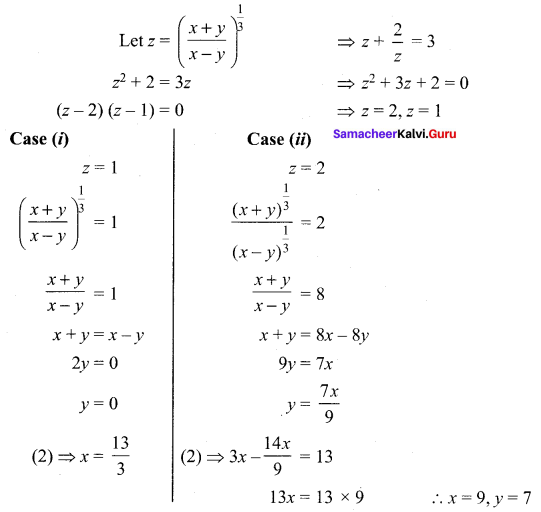Question 5.
5x – 1 + 51 – x = 26
Solution: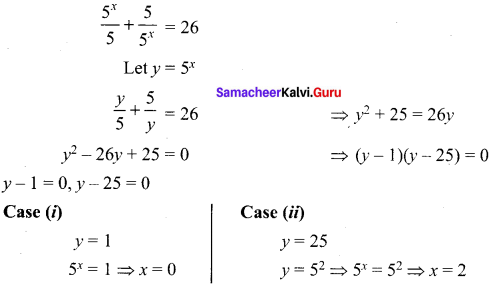Question 6.
Solve: 12x4 – 56x3 + 89x2 – 56x + 12 = 0
Solution:
Since the co-efficients of the equations are equal from both ends.
Divide the equation by x2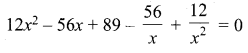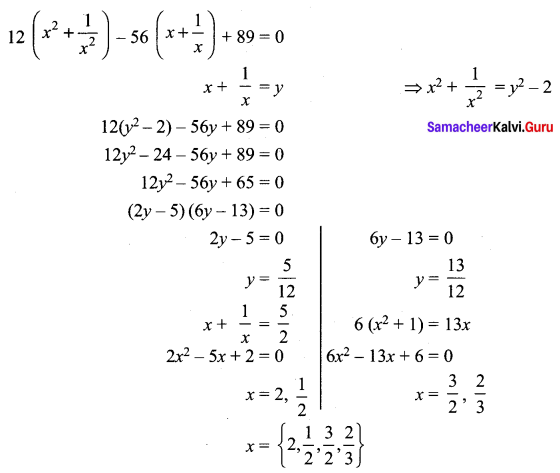Question 7.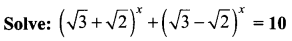Solution: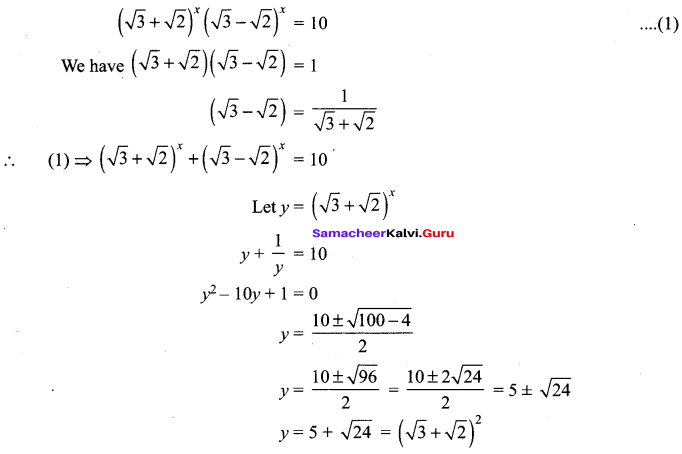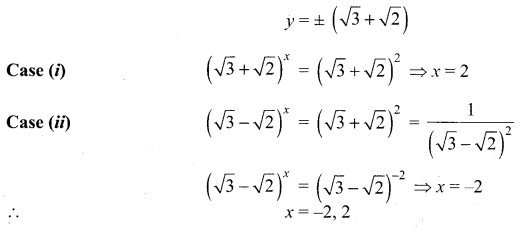Question 8.
Solve: x4 + 4x3 + 5x2 + 4x + 1 = 0
Solution: